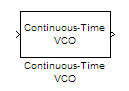Main Content

# Continuous-Time VCO

(To be removed) Implement voltage-controlled oscillator

Continuous-Time VCO will be removed in a future release. To design voltage-controlled oscillators (VCOs) and phase-locked loops (PLLs), use the Phase-Locked Loops (Mixed-Signal Blockset) blocks.

## Library

Components sublibrary of Synchronization

•## Description

The Continuous-Time VCO (voltage-controlled oscillator) block generates a signal with a frequency shift from the Quiescent frequency parameter that is proportional to the input signal. The input signal is interpreted as a voltage. If the input signal is u(t), then the output signal is

`$y\left(t\right)={A}_{c}\mathrm{cos}\left(2\pi {f}_{c}t+2\pi {k}_{c}{\int }_{0}^{t}u\left(\tau \right)d\tau +\phi \right)$`

where Ac is the Output amplitude parameter, fc is the Quiescent frequency parameter, kc is the Input sensitivity parameter, and φ is the Initial phase parameter.

This block uses a continuous-time integrator to interpret the equation above.

The input and output are both sample-based scalar signals.

## Parameters

Output amplitude

The amplitude of the output.

Quiescent frequency

The frequency of the oscillator output when the input signal is zero.

Input sensitivity

This value scales the input voltage and, consequently, the shift from the Quiescent frequency value. The units of Input sensitivity are Hertz per volt.

Initial phase

The initial phase of the oscillator in radians.

## See Also

### Blocks

Introduced before R2006a

## Support

#### Bridging Wireless Communications Design and Testing with MATLAB

Download white paper